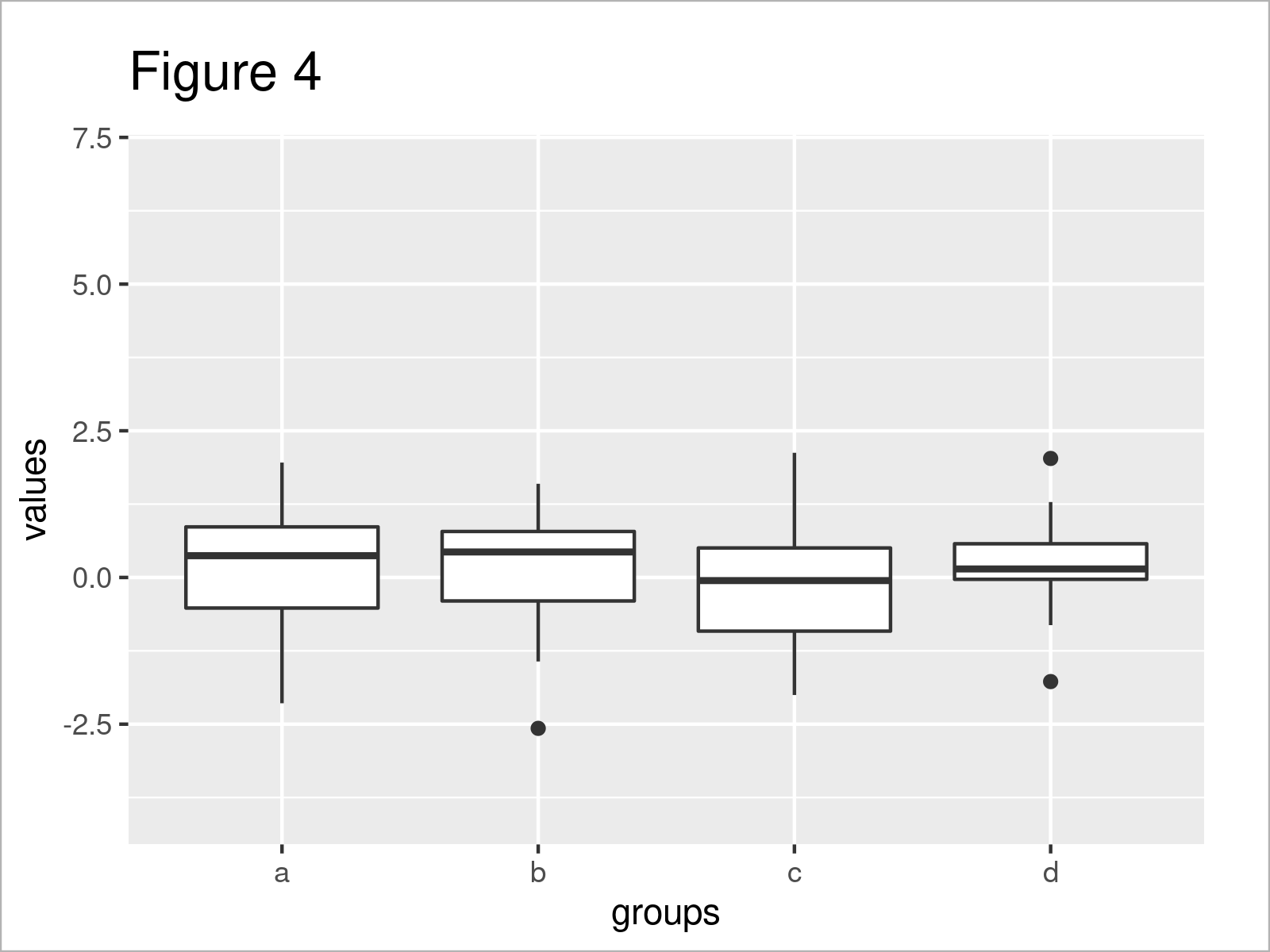# Change y-Axis Limits of Boxplot (Base R & ggplot2 Examples)

In this post you’ll learn how to set the y-axis limits of a boxplot in R programming.

The content is structured as follows:

So now the part you have been waiting for – the examples.

## Example Data

Consider the following example data:

```set.seed(328745)                # Create random example data frame
data <- data.frame(values = rnorm(100),
groups = letters[1:4])Table 1 shows the head of our example data: it is also visualized that our exemplifying data consists of two columns.

## Example 1: Change y-Axis Limits of Base R Boxplot

This example explains how to modify the y-axis scales of a box-and-whisker plot created with Base R.

Let’s first draw a Base R boxplot with default settings:

```boxplot(values ~ groups,        # Base R boxplot with default axes
data)```Figure 1 shows the output of the previous R code: A Base R boxchart with default axis limits.

If we want to change the axis limits of the y-axis, we can use the ylim argument and the c() function as shown below:

```boxplot(values ~ groups,        # Base R boxplot with manual y-axis
data,
ylim = c(- 4, 7))```By running the previously shown R programming syntax, we have managed to create Figure 2, i.e. a boxgraph with manually specified y-axis limits.

## Example 2: Change y-Axis Limits of ggplot2 Boxplot

The following code demonstrates how to create a ggplot2 boxplot with a manually changed y-axis.

We first need to install and load the ggplot2 software package, in order to use the functions that are included in the package:

```install.packages("ggplot2")     # Install & load ggplot2 package
library("ggplot2")```

In the next step, we can draw a default ggplot2 boxplot:

```ggp <- ggplot(data,             # ggplot2 boxplot with default axes
aes(groups,
values)) +
geom_boxplot()
ggp```As shown in Figure 3, the previous R syntax has created a ggplot2 boxplot with automatically defined axis scales.

We can now use the ylim function to adjust the limits of the y-axis:

```ggp +                           # Manual y-axis using ylim()
ylim(- 4, 7)```As shown in Figure 4, the previously shown R programming code has created a new ggplot2 boxplot with manually specified y-axis limits.

Alternatively to the ylim function, we may use the coord_cartesian function and the ylim argument:

```ggp +                           # Manual y-axis using coord_cartesian()
coord_cartesian(ylim = c(- 4, 7))```The output of the previous R code is illustrated in Figure 5 – You can see the same graphic as created by the ylim function.

## Video, Further Resources & Summary

Would you like to learn more about the specification of the y-axis limits of a boxplot? Then you might want to have a look at the following video instruction that I have published on my YouTube channel. In the video, I demonstrate the R programming code of this post:

Please accept YouTube cookies to play this video. By accepting you will be accessing content from YouTube, a service provided by an external third party.If you accept this notice, your choice will be saved and the page will refresh.

In addition, you may want to read some of the related tutorials on https://www.statisticsglobe.com/. I have released numerous tutorials on similar topics such as labels, ggplot2, colors, and graphics in R:

In this tutorial you have learned how to modify the y-axis scales of a boxplot graph in the R programming language. In case you have additional questions and/or comments, please let me know in the comments section.

Subscribe to the Statistics Globe Newsletter